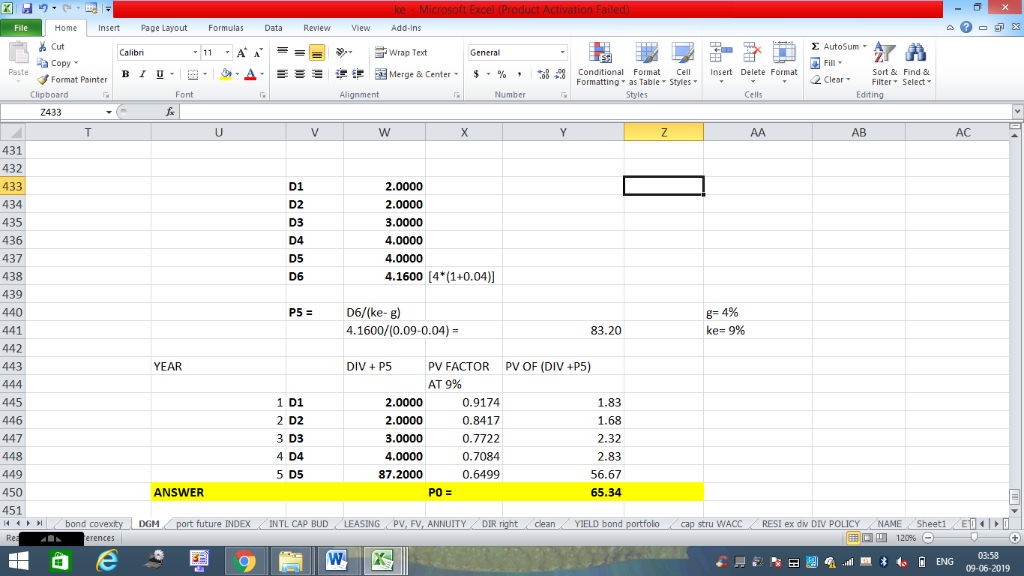In: Finance

# Variable Dividend Stock is expected to pay dividends of $2,$,2, $,3,$4, and $4 over... Variable Dividend Stock is expected to pay dividends of$2, $,2,$,3, $4, and$4 over the next five years. After that the dividend is expected to grow at a constant rate of 4%. If the return on the stock is 9%, what is the price?

Use formulas and show step by step with calculations.

## Solutions

##### Expert Solution## Related Solutions

##### (Stocks) A stock with a beta of 2.95 is expected to pay a $0.98 dividend over... (Stocks) A stock with a beta of 2.95 is expected to pay a$0.98 dividend over the next year. The dividends are expected to grow at 1.88% per year forever. What is the stock's value per share (to the nearest cent, no $symbol) if the risk-free rate is 0.32% and the market risk premium (i.e., the difference between the market return and the risk-free rate) is 5.86%? Note: You first need to find the required rate of return (r)... ##### (Stocks) A stock with a beta of 1.76 is expected to pay a$1.97 dividend over...
(Stocks) A stock with a beta of 1.76 is expected to pay a $1.97 dividend over the next year. The dividends are expected to grow at 2.38% per year forever. What is the stock's value per share (to the nearest cent, no$ symbol) if the risk-free rate is 0.58 and the market risk premium (i.e., the difference between the market return and the risk-free rate) is 6.27%? Note: You first need to find the required rate of return (r)...
##### Apple stock sells at $186.18 and is not expected to pay any dividend over the next... Apple stock sells at$186.18 and is not expected to pay any dividend over the next six months. The 6-month $180-strike call is selling at$27.90. The risk-free rate is 3% per annum continuously compounded. Options considered here are assumed to be of European style. a) What is the theoretical price of the 6-month put? b) The 6-month put is selling at $17.80 in the market. How would you undertake this arbitrage opportunity? Show the cash ﬂow table. c) How... ##### A stock is expected to pay the following dividends:$1.10 in 4 years, $1.70 in 5... A stock is expected to pay the following dividends:$1.10 in 4 years, $1.70 in 5 years, and$1.75 in 6 years, followed by growth in the dividend of 5% per year forever after that point. There will be no dividends prior to year 4. The stock's required return is 11%. The stock's current price should be $_________. * DO NOT ROUND INTERMEDIATE VALUES, NO CREDIT WILL BE GIVEN * FINAL ANSWER IN DOLLARS, ROUNDED TO TWO DECIMAL PLACES ##### A stock is expected to pay a dividend of$2 per share in three months. The...
A stock is expected to pay a dividend of $2 per share in three months. The share price is$75, and the risk-free rate of interest is 8% per annum with continuous compounding for all maturities. An investor has just taken a long position in a six-month forward contract on a share of stock. a) What are the forward price and the initial value of the forward contract? b) Three months later, immediately after the payment of the dividend, the...
##### A stock is expected to pay a dividend of €2 per share in 9 months. The...
A stock is expected to pay a dividend of €2 per share in 9 months. The stock price is €20, and the risk-free rate of interest is 5% per annum with continuous compounding for all maturities. An investor has just taken a long position in a 12-month forward contract on the stock. What is the Forward price (K)?
##### A stock is expected to pay the following dividends: $1 in 1 year,$1.5 in 2...
A stock is expected to pay the following dividends: $1 in 1 year,$1.5 in 2 years, and $1.8 in 3 years, followed by growth in the dividend of 7% per year forever after that point. The stock's required return is 14%. The stock's current price (Price at year 0) should be$____________. A stock is expected to pay the following dividends: $1.3 four years from now,$1.5 five years from now, and \$1.8 six years from now, followed by...